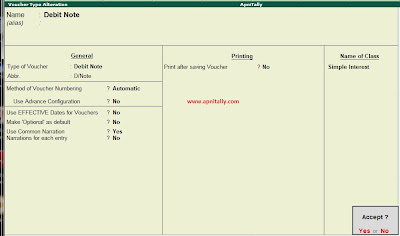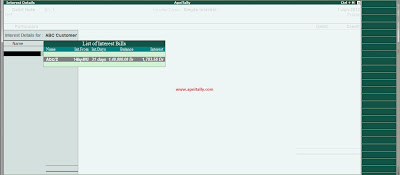# Tally Tutorial :How to Book Your Interest, Learn How to do it in Tally.ERP 9

In my, previous tutorial on Tally I have explained How to calculate Interest on your transactions. This is the part two and last part of this series. First of all you should learn how to calculate interest in Tally and then you should know how to book it in Tally.ERP9.
In the later part of that tutorial we have seen that Tally has the flexibility to automatically calculate the interest on time given by us and conditions given by us.
To continue the previous example we simply assume that a payment was made on July 31st and we have to calculate and book the interest on the amount till that date and accept the payment.
To do so we have to pass a debit note for the interest amount to book it. But to do it automatically we have to create a special class in Debit Note voucher. As we know that voucher class in Tally are for automate certain process so this class named Simple Interest is made to calculate the interest. So first of all alter the voucher type of Debit Note and create a class named Simple Interest.Simple Interest Class
Now put the option Use class for interest calculation to yes and accept the voucher class.Debit Voucher Alteration
Now pass the debit note voucher by selecting the class simple interest.Debit Note with voucher class
Choose the date you want and see that interest figure would automatically appear on the selection screen. Below are the screen shots of the interest calculated on different dates and the references to them as made by us.Interest as on June 1Interest as on July 31stInterest as on Aug 31st

As the payment of 2000000 has been made on July 31st so we would accept the payment but first book the interest upto July 31st. After debit voucher the ledger balance would be like this with added interest.Ledger Balance after Debit voucher
Now enter the receipt voucher. The bill by bill reference of the receipt  would look like this.Bill By Bill reference of receipt voucher.
Now you can see the pend ency of ABC Customer after the receipt. Here just go to Display -> Account books-> ledger and then press B for bill wise details. You would see that Rs. One lac is pending and the number of days due is 30. and Interest amount is due. Again when this due amount is paid then you pass a debit voucher on that date and settle the final amount.Pending Payment